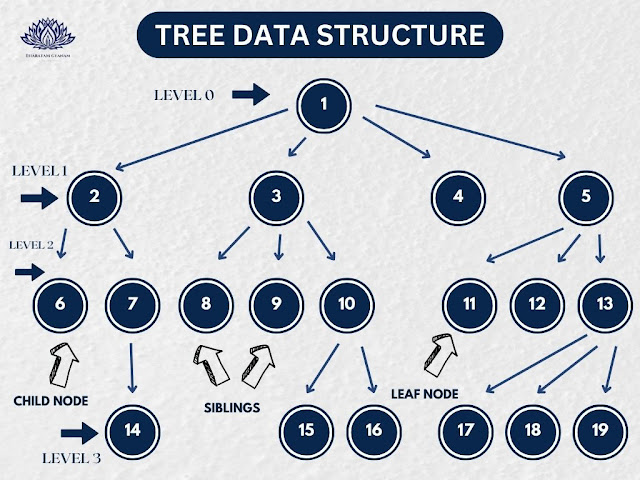# Tree Implementation in Data Structure - C++ Code

Trees are a fundamental data structure in computer science that are used to represent hierarchical relationships between objects. Trees are a collection of nodes, where each node has a value and a pointer to its child nodes. In this article, we will discuss trees in data structures in C++.Types of Trees:

There are various types of trees, but the most commonly used trees in computer science are:

Binary Trees: A binary tree is a tree in which each node has at most two children, left child and right child.

Binary Search Trees: A binary search tree is a binary tree where the left child of a node is less than or equal to the parent node and the right child is greater than or equal to the parent node.

AVL Trees: AVL trees are self-balancing binary search trees, which means that the height difference between the left and right subtrees of any node is at most one.

Red-Black Trees: Red-Black trees are another type of self-balancing binary search trees. They maintain balance using colorings on the nodes and a set of rules that ensure the balance is maintained.

B-Trees: B-trees are used to store large amounts of data on disk or other secondary storage devices.

Implementation of Binary Search Trees in C++:

We can implement binary search trees in C++ using classes and structures. Here is an implementation of a binary search tree in C++:

struct Node{
int data;
Node* left;
Node* right;
};

class BinarySearchTree{
public:
BinarySearchTree(){
root = NULL;
}

void insert(int data){
Node* newNode = new Node;
newNode->data = data;
newNode->left = NULL;
newNode->right = NULL;
if(root == NULL){
root = newNode;
return;
}
Node* current = root;
while(true){
if(data < current->data){
if(current->left == NULL){
current->left = newNode;
return;
}
current = current->left;
}
else{
if(current->right == NULL){
current->right = newNode;
return;
}
current = current->right;
}
}
}

void printInorder(Node* node){
if(node == NULL){
return;
}
printInorder(node->left);
cout << node->data << " ";
printInorder(node->right);
}

void printPreorder(Node* node){
if(node == NULL){
return;
}
cout << node->data << " ";
printPreorder(node->left);
printPreorder(node->right);
}

void printPostorder(Node* node){
if(node == NULL){
return;
}
printPostorder(node->left);
printPostorder(node->right);
cout << node->data << " ";
}

Node* search(int data){
Node* current = root;
while(current != NULL){
if(current->data == data){
return current;
}
else if(data < current->data){
current = current->left;
}
else{
current = current->right;
}
}
return NULL;
}

private:
Node* root;
};

In the above implementation, we have defined a structure called Node that has three fields: data, left, and right. data stores the value of the node, left is a pointer to the left child node, and right is a pointer to the right child node.

We have also defined a class called BinarySearchTree that has four methods:

1. insert(int data): This method inserts a new node with the given value in the binary search tree.

2. printInorder(Node* node): This method prints the values of the nodes in the binary search tree in inorder traversal.

3. printPreorder(Node* node): This method prints the values of the nodes in the binary search tree in preorder traversal.

4. printPostorder(Node* node): This method prints the values of the nodes in the binary search tree in postorder traversal.

5. search(int data): This method searches for a node with the given value in the binary search tree and returns a pointer to the node if found, otherwise it returns NULL.

In the insert method, we first create a new node with the given value and set its left and right pointers to NULL. If the tree is empty, we set the new node as the root node. Otherwise, we traverse the tree starting from the root node and insert the new node at the appropriate position based on its value.

In the printInorder, printPreorder, and printPostorder methods, we recursively traverse the tree and print the values of the nodes in the appropriate order.

In the search method, we traverse the tree starting from the root node and compare the value of each node with the given value. If we find a node with the given value, we return a pointer to that node, otherwise we continue traversing until we reach a leaf node or find the node with the given value.

Conclusion

Trees are a powerful data structure that are used in many applications in computer science. In this article, we discussed the different types of trees and implemented a binary search tree in C++. While the implementation shown here is simple, there are many optimizations and variations that can be made to improve its performance and functionality.